Journal topic
Solid Earth, 10, 969–985, 2019
https://doi.org/10.5194/se-10-969-2019Solid Earth, 10, 969–985, 2019
https://doi.org/10.5194/se-10-969-2019Method article 28 Jun 2019

Method article | 28 Jun 2019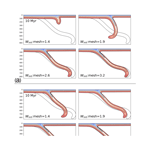# Improving subduction interface implementation in dynamic numerical models

Improving subduction interface implementation in dynamic numerical models
Dan Sandiford1,2 and Louis Moresi1 Dan Sandiford and Louis Moresi
• 1School of Earth Sciences, University of Melbourne, Melbourne, VIC, 3010, Australia
• 2Institute of Marine and Antarctic Studies, University of Tasmania, Hobart, TAS, 7004, Australia

Correspondence: Dan Sandiford (dan.sandiford@utas.edu.au)

Abstract

Numerical subduction models often implement an entrained weak layer (WL) to facilitate decoupling of the slab and upper plate. This approach is attractive in its simplicity, and can provide stable, asymmetric subduction systems that persist for many tens of millions of years. In this study we undertake a methodological analysis of the WL approach, and use these insights to guide improvements to the implementation. The issue that primarily motivates the study is the emergence of significant spatial and temporal thickness variations within the WL. We show that these variations are mainly the response to volumetric flux gradients, caused by the change in boundary conditions as the WL material enters and exits the zone of decoupling. The time taken to reach a quasi-equilibrium thickness profile will depend on the total plate convergence, and is around 7 Myr for the models presented here. During the transient stage, width variations along the WL can exceed 4×, which may impact the effective strength of the interface, through physical effects if the rheology is linear, or simply if the interface becomes inadequately numerically resolved. The transient stage also induces strong sensitivity to model resolution. By prescribing a variable-thickness WL at the outset of the model, and by controlling the limits of the layer thickness during the model evolution, we find improved stability and resolution convergence of the models.

1 Introduction

The process of stable asymmetric subduction requires that the down-going plate is substantially decoupled from the overriding plate along the subduction interface . Determining the combination of processes that allow plate-bounding faults to develop is a long-standing problem in geodynamics . Even when the subduction interface is assumed a priori, the implementation within a continuum modelling framework is not trivial. Accordingly, a range of modelling approaches have been developed. Different approaches may reflect the style of model (e.g. instantaneous vs. long term), the degree to which multiphysics are represented (e.g. hydrogeologic processes), the subduction driving forces (kinematic vs. dynamic), and numerical method (finite-difference vs. finite-element methods vs. boundary element methods). A common approach in long-term, dynamic models is to implement the subduction interface as a continuously entrained layer of weak material (WL). Figure 1a shows an example of this approach, implemented using Underworld2. This kind of approach is relatively simple to implement, and can provide stable, asymmetric subduction regimes that persist for many tens of millions of years, as shown in Fig. 1b. While the WL approach has been utilized in a broad range of studies, a detailed methodological analysis of this strategy is lacking (although valuable insights appear in ). This study focuses on the behaviour of a constant-viscosity WL implementation, within a 2-D thermo-mechanical subduction setup. The results have relevance for the precision, efficiency, and reproducibility of dynamic subduction models.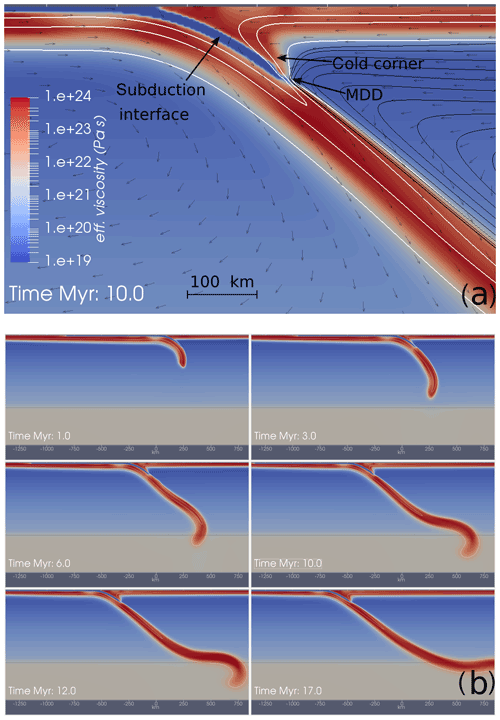Figure 1Example of dynamic subduction simulation. Colour map shows the effective viscosity on a logarithmic scale, and reveals the weak finite-width subduction interface which enables the decoupling of the slab and the upper plate. The implementation of the interface follows a modified WL approach, which we refer to as an embedded fault (EF). The subduction interface extends to 100 km depth, beyond which the slab and mantle become coupled (MDD). Above the MDD, part of the mantle wedge tends to become stagnant due to the strong temperature dependence of the rheology. This is often referred to as a cold corner. (a) Closeup of slab in the upper mantle. White contour lines show 400, 700, and 1200 C isotherms. Black lines are streamlines of the velocity in a fixed upper plate reference frame. Arrows show model velocity field. (b) Time evolution of the same model, showing slab rollback and interaction with the transition zone.

2 The subduction interface

The subduction interface refers to the plate boundary fault at Earth's convergent margins. Exhumed subduction interfaces are typified by melange zones, often hundreds of metres in width, with coherent blocks embedded within a sedimentary and/or serpentinized matrix . The subduction interface zone is characterized by rheological and petrological complexity, low strength, and the abundance of water and the critical role of fluid pressure . Subducted sediments can reach depths of up to 80 km , similar to the inferred depth at which the slab and mantle become coupled, and can be traced in the composition of arc magmas . The proportion of subducted sediment may lead to large variations in the mechanical properties in the deep subduction interface . Entrainment of upper plate material in a process known as subduction erosion also occurs at a substantial number of convergent margins . Subduction interfaces therefore incorporate material from the subducting plate, the accretionary prism, and the upper plate . However, the degree to which variability in these influxes impacts long-term subduction dynamics is debated .

Fluid pressure plays a key role in subduction zone interfaces as it determines the effective frictional strength, controls the seismic/aseismic character of slip , and dictates the extent of hydrous mineral formation along the subduction interface (Reynard2013). In the shallow megathrust, pore fluid water and clay minerals are thought to exert a major control on the rheology (Vrolijk1990). Seismic imaging demonstrates the presence of fluids along the plate boundary. In some cases pore pressures are inferred to be near lithostatic values . While many subduction zone megathrusts are capable of hosting great earthquakes, there is a spectrum of behaviour from locked to creeping. Ongoing debate surrounds the strength implications of this divergent behaviour . The limit of the seismogenic zone can range from 5 to 50 km in depth . The topography of most forearcs is consistent with average shear stresses of 15 MPa over the long term (Lamb2006). Megathrust shear stresses in the range 1–100 MPa, with mean shear stresses also around 15 MPa, are consistent with results from heat flow studies .

At greater depths, fluids are generated by devolatilization reactions . The deeper part of the subduction interface where the slab is in contact with the serpentinized upper plate mantle is thought to be controlled primarily by weak hydrous minerals which allow effective slab–mantle decoupling but also inhibit unstable seismic slip . It remains unclear whether the rheology of the deep subduction interface is dominated by viscous or (stable) plastic behaviour . The thickness of subduction interfaces, particularly in the deeper aseismic zone, is not well constrained. Estimates range from between tens of metres to a few kilometres . used structural relationships in exhumed subduction interfaces to infer the relative strength of different lithologies. Metasediments and serpentinites which commonly form the matrix within the subduction melange appear to be the weakest components.

3 Past modelling approaches

In efforts to study subduction zone dynamics, a range of modelling approaches have been developed. The subduction interface is a necessary model component whenever both subducting and upper plates are included. One approach is to incorporate the interface directly as a kinematic constraint into the simulation, i.e. by specifying continuous normal and discontinuous tangential velocities in the model solution surrounding the fault . This idea was developed into a dynamic framework with finite-element models that incorporate internal stress boundaries; the result is zero width faults within the continuum mechanical representation of the lithosphere . Because moving mesh nodes are needed to capture proper fault advection, accurate tracking of large-scale deformation is challenging. A more common approach is to apply finite regions of weak constitutive behaviour within a static mesh. The velocity field naturally develops strain localization around the weak zone, although the “faults” are usually much broader than real plate boundaries. This approach was first applied as a spatially fixed low-viscosity zone that could decouple the plates but would not allow trench motion (e.g. Gurnis and Hager1988). This type of implementation was developed so that narrow low-strength weak zone “stencils” could also be advected to allow trench motion .

Over the past decade the use of an entrained weak layer (WL) has become an increasingly common strategy . Rather than using a fixed weak zone, the subduction interface is typically implemented by imposing a material layer at the top of the subducting plate that is advected with the flow and continuously entrained into the decoupling region. The WL approach enables a mobile trench while also helping to accommodate plate bending, which is important in the absence of a free surface. Additionally, it isolates the extremely weak parts of the system (the plate boundary) from the plates, enabling long-term asymmetric subduction to occur. In this study, we focus on a simple constant-viscosity implementation of the WL using the Underworld2 code, as described in Sect. 2.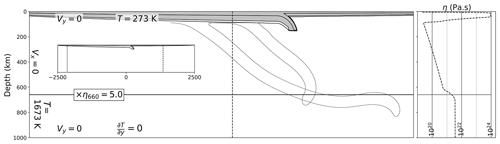Figure 2Initial conditions for 2-D thermo-mechanical subduction models. All models in this study have a 50 Myr initial slab age. Velocity boundary conditions are free slip on all walls of the domain (zero tangential stress). The dark grey region in the main panel shows parts of the model colder than 1100 C. The inset shows the full domain. Outlines show the slab position at 10 and 20 Myr. The right-hand panel shows the viscosity profile evaluated along the vertical dashed line in the main panel.

Although the WL is commonly implemented in numerical subduction models, there is some ambiguity as to whether this approach represents an attempt to explicitly model subduction interface dynamics, or should instead be conceived as an ad hoc solution to modelling the plate boundary. WL implementations do mimic several critical processes that are thought to contribute to the long-term weakness and stability of the subduction interface. One of these is the process of entrainment or “self-lubrication” . The entrainment of both sediments and fluids along the slab interface likely plays a key role in maintaining subduction interface weakness. Additionally, the WL approach might be linked with deformation-localizing processes such as damage, grain size reduction, and fabric development. Indeed, WL models have been conceived as a limiting case in which comprehensive damage is assumed within the interface material and hence can be prescribed at the outset . Despite the fact that we can draw analogies to relevant physical processes, our view is that the WL approach constitutes an ad hoc solution to the sub-grid phenomena of plate boundary shear localization.

The behaviour of the WL is discussed is some detail in . These studies highlight the tendency for the subduction interface to develop spontaneous thickness variation as the models evolve. Typically the interface widens near the trench, building a prism-like complex, and thins at depths beyond the brittle–ductile transition (>50 km). This pattern was also noted in the boundary element models of , who attributed a downdip thickness variation to lubrication layer dynamics. The tendency for thinning will impact the level of mesh resolution required in a model (Arcay2017). Additionally, WL formulations are likely to evolve maximum subduction interface thickness around twice the imposed thickness, potentially impacting effective stress along the interface, as well as the accuracy of predicted thermal structure. Understanding and controlling these spontaneous width variations will be important in terms of using dynamic models to explore sensitive subduction zone processes, such as metamorphism and melting near the slab top.

4 Methods

## 4.1 Numerical model setup

The numerical subduction models developed in this study represent the time evolution of simplified conservation equations for mass, momentum, and energy within a 2-D Cartesian domain. Figure 2 provides an overview of the model domain, as well as initial and boundary conditions. The depth of the domain is 1000 km, and the aspect ratio is 5. Initial temperature conditions define two plates which meet at the centre of the domain, including a small asymmetric slab following a circular arc to a depth of 150 km. The subducting plate has an initial age of 50 Myr at the trench, while the upper plate age is 10 Myr. Both plates have a linear age profile with an initial age of zero at the sidewalls. This setup allows the model to evolve under the driving force of internal density anomalies (sometimes referred to as a fully dynamic model). Apart from the presence of a WL, there is no compositional difference between the subducting and upper plate, nor do we include any compositional differentiation within the oceanic lithosphere. The only aspects of the model setup that are varied are the details of subduction interface implementation (described in the following section) and the model resolution.

The mantle is treated as an incompressible, highly viscous fluid in which inertial forces and elastic stresses can be neglected. The mechanical behaviour of mantle (including the thermal lithosphere) is prescribed by a composite rheological model that includes a linear high-temperature creep law, as well as a scalar visco-plastic flow law, sufficient for capturing pseudo-brittle as well as distributed plastic deformation within the slab. Thermal buoyancy is the only source of density variation in the model. The thermal variations are coupled to the momentum equation through their effect on density, which follows the Boussinesq approximation. A detailed description of the governing equations, constitutive laws, and physical parameters is given in Appendix A.

Approximate solutions to the incompressible momentum and energy conservation equations are derived using the finite-element code Underworld2. Underworld2 is a Python API which provides functionality for the modelling of geodynamic processes. Underworld2 solves the discrete Stokes system through the standard mixed Galerkin finite-element formulation. The domain is partitioned into quadrilateral elements, with linear elements for velocity and constant elements for pressure (Q1∕dP0) . Material properties are advected on Lagrangian tracer particles. Unless otherwise stated, models have a mesh resolution of 160 elements in the vertical direction, refined to provide an element width of ∼3 km at the surface, and a particle density of 30 tracers per element. Particles are added and removed to maintain density near this value. During quadrature, material properties are mapped to quadrature points using nearest-neighbour interpolation. The Lagrangian tracer particles are used to distinguish the subduction interface material from the rest of the system (lithosphere and mantle). Underworld2 solves the energy conservation equation using an explicit streamline upwind Petrov–Galerkin (SUPG) method . In this approach, a Petrov–Galerkin formulation is obtained by using a modified weighting function which affects upwinding-type behaviour. The Stokes system has free-slip conditions on all boundaries. The energy equation has constant (Dirichlet) and zero-flux (Neumann) on the top and bottom boundaries respectively. The left and right sidewalls have a constant temperature equal to the mantle potential temperature (1673 K). The surface temperature is 273 K.

## 4.2 Subduction interface implementation

The focus of our study is a common approach to modelling the subduction interface, sometimes referred to as a weak layer or weak crust. This approach has two intrinsic features: (1) the material that provides decoupling within the interplate zone is a distinct material type with rheology that contrasts with the plates/background material, and (2) the weak material layer is distributed along some or all of the subducting plate, so that the flow itself entrains new weak material into the decoupling region . In this study we use the abbreviation WL to refer to a typical implementation, namely one in which the distribution of weak material is fully self-evolving within the deforming subduction interface zone. We also demonstrate a modified version of this approach, which we refer to as an embedded fault (EF). The EF implementation differs from the WL in that the width of the interface is constrained in terms of its minimum and maximum thickness. While this manipulation of the interface material could be achieved in different ways, our implementation is based on a reference line of particles, which lie at the base of the weak interface and are advected along with the material swarm. At each time step we remap material types based on the relative position of the material particles with regard to the reference line.

The relationship between the EF reference line, the material swarm, and the mesh is shown in Fig. 3. In the EF approach, we also initialize the interface with a non-uniform thickness. For reasons discussed in the following sections, the thickness of the interface material within the decoupling zone should be close to double the thickness that is prescribed on the top of the subducting plate.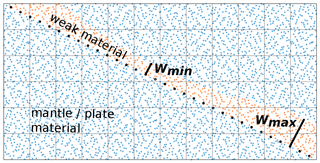Figure 3Schematic of the embedded fault (EF) implementation. The EF is a modification to the standard weak layer approach (WL). As with the WL, shear localizes due to a finite-width layer of weak material (represented by orange particles). A reference line of tracer particles (black points) is advected with the flow, at the base of the weak material layer. This line provides a reference to enforce width limits on the weak material, denoted by Wmin and Wmax. At each time step we remap material types based on the relative position of the material particles to the reference line. Note that the element size here is not shown to scale.

It is important to emphasize that differences between the WL and EF implementations relate only to the distribution of weak material within the model. All other aspects of the interface representation remain identical. In each case the interface has a constant viscosity. Weak material is continually prescribed along the uppermost part of the subducting plate as it moves away from the ridge, with a specified constant thickness (Winit=10 km). The upper plate does not contain any weak or interface material. For simplicity, there is no rheological variation between the shallow (frictional) megathrust and the deeper, viscous interface. To control the upper limit of the MDD, a depth-dependent cosine taper is used to transition the subduction interface rheology to the background mantle rheology. The taper for the transition (both WL and EF) begins at 100 km, and has a width of 30 km. Decoupling is strongly inhibited at depths greater than the taper onset. Hence the depth of the taper onset (100 km) effectively controls the upper limit of MDD. Likewise, at a distance of 800 km from the trench, along the subducting plate, the rheology of the interface material transitions to background mantle rheology. This avoids interaction of weak material with the spreading ridge.

5 Analysis of modelling approaches

## 5.1 The weak layer approach

Figure 4 shows the evolution of the subduction interface thickness over a 20 Myr period, based on the WL setup described in Sect. 4. This model reveals the spontaneous development of substantial WL thickness variations, not only near the trench (i.e. near the accretionary prism) but throughout the entirety of the decoupling zone. Previous studies have commented on the occurrence of similar thickness variations , although their origin has not been closely considered. We propose that such variations arise primarily from the way that the kinematics of the flow in the WL effect the downdip volumetric flux. In the following discussion we refer to a slab-based coordinate system in a fixed upper plate reference frame, where $\stackrel{\mathrm{^}}{y}$ is the direction orthogonal to the slab midplane, $\stackrel{\mathrm{^}}{s}$ is the direction parallel to the slab midplane, and the plate convergence velocity is equal to the subducting plate velocity (Vs). Before reaching the trench, the WL material travels with the subducting plate, with near-uniform velocity and negligible shear ($\frac{\partial {V}_{\mathrm{s}}}{\partial \stackrel{\mathrm{^}}{y}}\approx \mathrm{0}$). In the subduction interface zone, however, the WL material decouples the slab and upper plate, and velocity gradients across the interface are finite. For a Newtonian rheology, the gradient is expected to be linear with $\frac{\partial {V}_{\mathrm{s}}}{\partial \stackrel{\mathrm{^}}{y}}\approx \frac{{V}_{\mathrm{s}}}{W}$ (i.e. Couette flow). This change in velocity profile across the WL means that there is a smaller volumetric flux (per unit length normal to the interface) compared to the flux of material being delivered on the incoming subducting plate. To illustrate this point we consider a simple boundary-driven Stokes flow, as shown in Fig. 5, which provides a useful analogy to the flow in the WL. In this model, flow is driven by a constant tangential velocity on the lower boundary, while the upper boundary of the model has a patch of frictional (no slip) nodes, surrounded on both sides by a free-slip boundary condition. The step change in boundary conditions imposes volumetric flux gradients causing the weak layer (shown in blue) to thicken near the start of the no-slip region, and thin near the end. The width changes in the weak layer proceed until the overall volume flux reaches equilibrium.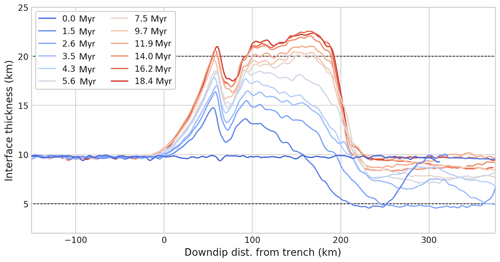Figure 4Interface thickness evolution in WL implementation. The initial layer thickness (Winit) is 10 km. Coloured lines show the evolving thickness plotted as a function of downdip distance from the trench. Dashed black lines show the expected maximum (equilibrium) and minimum (transient) thickness, based on a kinematic description of the flow evolving from a uniform thickness weak layer.

Returning to our subduction model results, the validity of our description can be tested by considering the thickness profile required for volume flux equilibrium. Assuming that the decoupling zone maintains a Couette profile, independent of the local thickness, flux equilibrium requires that the thickness of the WL in the decoupling zone be twice that imposed on the incoming plate. This prediction, based on a simplified kinematic description of flow in the interface, is broadly consistent with the long-term evolution observed in the WL models (e.g. Fig. 4).

While our discussion so far has mainly emphasized the transition of the WL from the oceanic part of the subducting plate into the decoupling zone, similar processes take place where the slab and the mantle become coupled (the MDD). When a constant interface width is prescribed, the interface thickness near the MDD initially decreases. Again, this is because the volumetric flux increases as Couette flow in the decoupling region transitions to the fully coupled flow. The location where the subduction interface thins provides a proxy for the MDD. Complexity arises due to the fact that the MDD in our model is not constant. Instead the MDD tends to become deeper as the corner of the mantle wedge cools and stagnates. This means that the effective boundary conditions on the flow within the interface are also time varying. This accounts for why the location of interface thinning migrates downdip as time progresses, as shown in Fig. 4.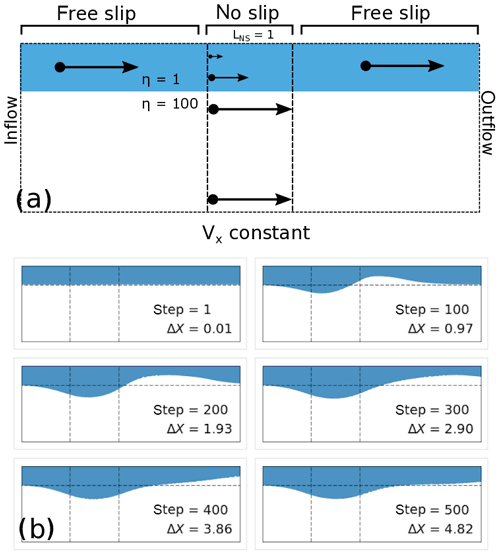Figure 5Analogue for WL thickness evolution. (a) Model setup for a boundary-driven flow where a weak layer (shown in blue) interacts with a stronger layer (white). The top surface of the model is free slip, except for a patch of no-slip nodes (Vx=0). The bottom surface has a constant horizontal velocity component. (b) Evolution of material in the boundary-driven model. ΔX represents the accumulated displacement along the bottom boundary, normalized by the width of the no-slip patch.

Based on analysis of the typical WL setup, we argue that the primary thickness variations reflect a simple response to changes in volumetric flux along the interface. In addition to these flux-controlled changes, Fig. 4 shows that the subduction interface also develops a persistent short-wavelength width perturbation just beneath the tip of the forearc at a downdip distance of ∼60–80 km. Here the interface thins by ∼3–4 km. This occurs in combination with strong, localized plastic deformation in the upper plate.

It seems likely that such short-wavelength anomalies may be affected by a range of factors, including the interface and lithosphere rheology, mesh resolution, and material advection scheme. If so, these features are likely to be somewhat model dependent, in contrast to the long-wavelength, flux-related thickness variations which reflect intrinsic kinematics of flow in the WL setup.

## 5.2 Improving the weak layer approach

The embedded fault (EF) implementation, described in Sect. 4, consists of two complementary strategies. Firstly, we may initiate the WL with a variable thickness. Secondly we can control the WL thickness throughout the simulation, by remapping WL material (particles) to background material, and vice versa. Given these controls, perhaps an obvious first issue to address is what happens if we simply enforce a constant width interface at all times. While this would in some ways be a desirable approach, doing so results in the development of a very spurious subduction morphology. Figure 6b shows results from such a case (${W}_{\mathrm{max}}={W}_{\mathrm{min}}={W}_{\mathrm{init}}=\mathrm{10}$ km). After 15 million years of model evolution a very atypical subduction morphology has developed, with an extremely low-angle megathrust beneath a forearc region with a width of greater than 600 km. While geologically irrelevant, the example shown in Fig. 6b provides a useful insight into the way in which the flow in the interface can influence model dynamics. When the subduction interface is forced to remain at constant width, the interface is unable to evolve towards flux equilibrium. Persistent interface-normal velocity components result, and the compounding effect eventually distorts the morphology of the entire subduction hinge region.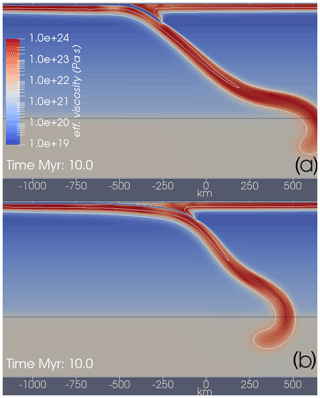Figure 6Effect of variable maximum Wmax in EF models. The colour map shows the effective viscosity at a model time of 10 Myr. White lines show isotherms at 700 and 1200 C. Panel (a) shows the EF model with ${W}_{\mathrm{max}}=\mathrm{1.9}×{W}_{\mathrm{init}}=\mathrm{19}$ km. Panel (b) shows the EF model with ${W}_{\mathrm{max}}={W}_{\mathrm{init}}=\mathrm{10}$ km. In this case, due to the fact that the subduction interface cannot adjust its thickness, an extremely long, low-angle interface develops, with forearc distances of ∼500 km.

Figure 6a shows the slab morphology developed by instead using ${W}_{\mathrm{max}}=\mathrm{1.9}×{W}_{\mathrm{init}}=\mathrm{19}$ km (we discuss the choice of 1.9 in the next section). The subduction morphology in this simulation is more realistic, and consistent with outcomes from a standard WL approach. The evolution of the interface thickness from the same simulation is shown in Fig. 8. In addition to controlling the width of the interface throughout the EF simulation, we have also prescribed the initial interface thickness in the decoupling zone (from the trench to a depth of 100 km) to have value equal to Wmax (19 km). In this way, we have tried to preemptively impose a thickness profile closer to the flux equilibrium. Figure 8 shows that this strategy reduces, but does not fully eliminate, the transient stage of interface adjustment. It is difficult to fully eliminate the transient stage because the equilibrium interface thickness profile is partly determined by the MDD, which is not constant. The evolution of the MDD is a response to the cooling of the mantle wedge and the development of a stagnant “cold corner” (see Fig. 9). Prescribing initial temperature conditions that include the cold corner would be one way to further reduce the amount the transient adjustment of the interface. Figure 7 shows the state of the material swarm in the EF model, compared to the equivalent WL model. This reveals a typical distribution of interface material once a quasi-equilibrium thickness has been established.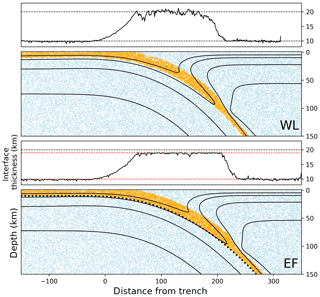Figure 7Distribution of subduction interface material. Results from a standard WL model are shown in the top panel. The EF model is shown in the bottom panel. Both models have a constant viscosity interface rheology. Model time is 12.5 Myr in both cases. In the lower panels, orange points are the subduction interface material, and blue points are the background material (mantle/lithosphere). Points in the material swarm and along the EF reference line have been down-sampled for clarity. Solid black lines over the material points are isotherms. Smaller panels show the measured interface thicknesses in each case; red horizontal lines show the thickness constraints Wmin, Wmax.

In addition to the thickness variations related to volumetric flux, the WL approach can develop short wavelength thickness variations, as seen in Fig. 4. In the EF implementation, we found that using a value of Wmax slightly less than 2.0×Winit helps to suppress short wavelength thickness variations, without significantly effecting the overall model evolution. In other words, while we need to allow the interface to develop some amount of thickness variation, it may be advantageous to use a value slightly less than Winit. Figure 10 shows results from a number of experiments where the value of Wmax was changed. Qualitatively, we see that the models have very similar long-term evolution once Wmax is greater that 1.75×Winit.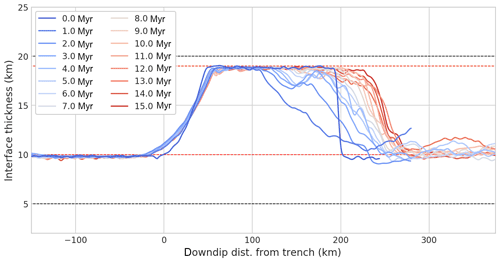Figure 8Interface thickness evolution, EF approach. In the EF implementation limits are imposed in the maximum and minimum thicknesses, as shown by the red dashed lines. Unlike the standard WL approach, the initial thickness of the interface is variable. The initial thickness of the interface within the decoupling region is equal to Wmax. The black lines show the typical range in which a WL model will vary, assuming a constant initial thickness of 10 km (see also Fig. 4). The results shown here refer to the same model as shown in Figs. 1 and 6.

## 5.3 Stability and convergence

So far we have discussed results based on well-resolved models, with 160 elements across the 1000 km vertical domain. At this resolution, the vertically refined mesh provides 3.2 elements within the subduction interface (at Winit=10 km). Note that this increases to more than six elements in the decoupling zone, once the interface has thickened to ∼20 km. We now look at the convergence of models under variable resolution, based on a standard WL approach as well as the EF implementation. Figure 11 shows the slab temperature field at 10 Myr for a set of models with varying resolution: 72, 96, 128, 160, and 192 elements in the vertical dimension. The ratio of the initial interface width (Winit) to the element width has values of 1.4, 1.9, 2.6, 3.2, and 3.8 for the respective mesh resolutions. The dashed slab outline in Fig. 11 shows the morphology for a simulation with 192 elements in the vertical dimension; this is used as a reference model in the following analysis. Note that while we have varied the mesh resolution, all models have the same spatial particle density. Figure 11a shows results using a standard WL implementation. At the lowest resolution (72 elements) the simulation stalls and the slab undergoes runaway thermal decay. At 96 elements, the model is still strongly impacted by under-resolution of the subduction interface. Figure 11b shows equivalent results using the EF implementation. Qualitatively, we see that EF models are more stable at lower resolution. For instance, the EF model at the lowest resolution (72 elements) more closely reproduces the evolution of the reference model than does the WL model with 96 elements.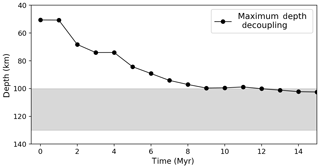Figure 9Evolution of the maximum depth of decoupling (MDD). As the mantle wedge cools, it progressively stagnates, driving deeper decoupling within the subduction interface. The grey region in the figure shows the depth interval over which the subduction interface transitions to the background mantle rheology as prescribed with a cosine taper (see Sect. 4). Results are from the same model as shown in Fig. 6a, i.e. an EF model with ${W}_{\mathrm{max}}=\mathrm{1.9}×{W}_{\mathrm{init}}$.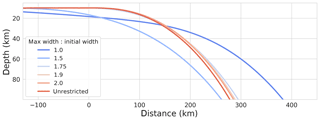Figure 10Embedded fault models (EF) with variable Wmax. Lines show the morphology of the base of the subduction interface (the EF reference line) at 10 Myr for different models with varying Wmax. When the interface thickness variation is strongly constrained, the evolution of the model is significantly effected. See also Fig. 6.

Figure 11 suggests that the EF models converge more closely with increasing resolution. We quantify this by tracking the relative error (L2) of the temperature field in the lower-resolution models with respect to the reference model (192 elements). The relative error with respect to the reference model (Tref) is

$\begin{array}{}\text{(1)}& E={\left[\frac{{\int }_{\mathrm{\Omega }}\left(T-{T}_{\mathrm{ref}}\right)\cdot \left(T-{T}_{\mathrm{ref}}\right)\mathrm{d}V}{{\int }_{\mathrm{\Omega }}{T}_{\mathrm{ref}}\cdot {T}_{\mathrm{ref}}\mathrm{d}V}\right]}^{\mathrm{1}/\mathrm{2}}.\end{array}$

Figure 13 shows relative error results for the same set of models shown in Fig. 11, confirming more rapid resolution convergence in the EF models relative to the standard WL approach. For most of the models shown in Fig. 13 the relative error accumulates rapidly in the first 7–10 million years of the simulation, while the error rate flattens after this. This is similar to the time taken for the WL interface to reach its equilibrium thickness (e.g. Fig. 4). This suggests that models are strongly resolution-sensitive during the transient phase of the interface adjustment. At low resolution (72, 96, 128 elements), errors in both WL or EF models express this sensitivity. Interestingly, at 160 elements, the EF case exhibits nearly constant error accumulation during the model evolution. This suggests that we have succeeded in reducing the sensitivity of the subduction interface implementation, relative to the overall error accumulation rate. The latter may be influenced by additional factors which we have not controlled for here, such as the time step size in the advection–diffusion implementation which is controlled by the mesh resolution.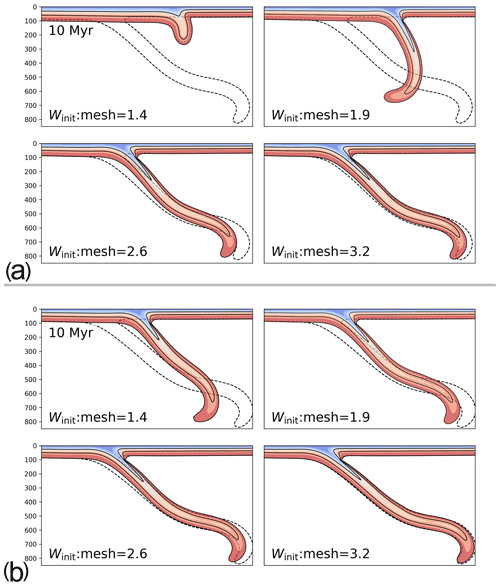Figure 11Implementation comparison with variable resolution. Colour map shows slab temperature field at 10 Myr, masked above 1250 C. Each figure shows a series of models run at different resolutions. The total number of elements in the vertical coordinate was 96, 127, 160, and 192. Corresponding subduction interface resolution is displayed in the figure, representing the initial fault thickness (Winit) divided by the local element size. (a) Models using standard WL implementation. (b) Models using the EF implementation.

Figure 12 shows the distribution of interface material in the lowest-resolution WL model (shown in the top left panel in Fig. 11a). In this case, under-resolution of the weak layer induces strong coupling between the slab and the upper plate at relatively shallow depths and begins to thin the WL, due to the development of strong flux gradients (as described in Sect. 5). This induces further coupling and yet more thinning. This proves to be catastrophic feedback process, causing the simulation to stall and enter runaway thermal decay. The EF approach provides stability in this context, by inhibiting the feedback cycle. While this behaviour is mainly relevant for models run at low resolution, the increased stability of the EF approach is a useful property, particularly from a model development perspective.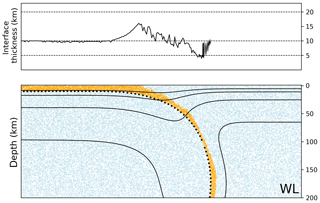Figure 12Interface thickness in under-resolved WL model. In the lower panel orange points show the subduction interface material, and blue points are the background material (mantle/lithosphere). The upper panel shows interface thickness. This figure shows the model state 10 Myr after model initiation. This model is also shown in the upper left panel of Fig. 11a.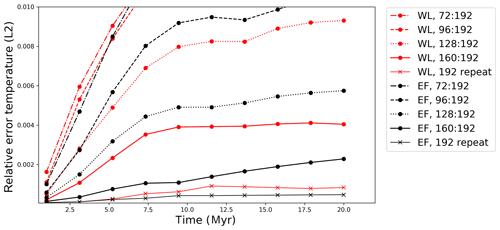Figure 13Convergence of models with varying resolution. Vertical axis shows the relative error (L2) of the temperature field. We have truncated the vertical axis so as to focus on trends in the higher-resolution results. The error value is based on the error between the highest-resolution model (192 elements in the vertical axis) and each of the models with lower resolution, as labelled in the figure. Experiments were repeated at the highest resolution to provide a baseline for model reproducibility (labelled “repeat”).

6 Discussion and conclusions

The entrained weak layer (WL) is a common approach for implementing the subduction interface in long-term dynamic simulations. We have discussed aspects of WL implementation that can have an unintended impact on model evolution. The first involves the transient evolution of a uniform thickness interface to a variable thickness–uniform flux configuration. If not properly accounted for, thinning of the deeper part of the WL could lead to numerical under-resolution, as previously suggested (Arcay2017). If the WL has a viscous rheology, the thinning will lead to higher stresses. This can induce a positive feedback when higher stresses increase the amount of partial coupling, inducing further thinning. Even for seemingly well-resolved models, the transient behaviour of the subduction interface appears to be responsible for strong mesh sensitivity and poor resolution convergence. In general, models with plastic/frictional rheologies should be less sensitive to these transient adjustments, as the stress should not depend on the width of the interface.

Another tendency of the WL models is to develop persistent short-wavelength thickness variations. These may represent interface instabilities, as are observed in Couette flows past a deformable boundary . These tend to dominate on the shallow part of the boundary with the upper plate. While flux-related thickness variation will be expected for any model implementation of WL, boundary instabilities (short wavelength) are likely to be more variable across different codes. They may depend on additional details of implementation, such as the rheology of the plates, material advection, and interpolation schemes. Together, these issues are likely to hinder efforts to produce reproducible results between codes.

These unintended behaviours of the WL approach can be partly mitigated by controlling the thickness of the interface. We demonstrate a simple implementation of this concept, which utilizes a line of reference points at the base of the WL, allowing us to remap material types based on proximity to this line. We call this approach an embedded fault (EF). The ability to constrain the thickness of the interface improves the resolution convergence of numerical models, as well as the stability at lower resolutions. The EF does add complexity to models, in both the sense of implementation as well as the introduction of new parameters to control specific details (e.g. Wmax). There are obviously other implementation strategies that could be developed in order to achieve similar outcomes. These should be explored in future studies. Overall, this study provides a better understanding of the behaviour of subduction models utilizing WL approaches. These insights offer a basis for achieving better outcomes in terms of model reproducibility and precision.

Code and data availability
Code and data availability.

The scripts used to run these models, as well as data and the output of the simulations, are accessible on request. The scripts are compatible with a Docker version of the Underworld2 code, available at https://hub.docker.com/r/dansand/underworld2-dev/ (last access: 15 April 2019). The Underworld2 code is open-access and available at https://github.com/underworldcode/underworld2 (last access: 15 April 2019).

Appendix A: Governing equations, constitutive relationships, and physical parameters

## A1 Continuity, momentum, and energy equations

On geological timescales the Earth's mantle behaves as a highly viscous, incompressible fluid, in which inertial forces can be neglected. The flow caused by internal buoyancy anomalies is described by the static force balance (momentum conservation) and continuity equations:

$\begin{array}{}\text{(A1)}& {\mathit{\sigma }}_{ij,j}+\mathit{\rho }{g}_{i}=\mathrm{0},\text{(A2)}& {u}_{i,i}=\mathrm{0},\end{array}$

where ui is the ith component of the velocity. Repeated indices denote summation, and u,i represents partial derivative with respect to the spatial coordinate xi. The full stress tensor appearing in Eq. (A1) can be decomposed into deviatoric and mean components:

$\begin{array}{}\text{(A3)}& {\mathit{\sigma }}_{ij}={\mathit{\tau }}_{ij}+p{\mathit{\delta }}_{ij}.\end{array}$

It is noted that sign of the pressure (p) is opposite to the mean stress tensor, consistent with the convention that fluid flows from high to low pressure. The deviatoric stress tensor (τ) and the strain rate tensor (Dij) are related according to the constitutive relationship:

$\begin{array}{}\text{(A4)}& {\mathit{\tau }}_{ij}=\mathrm{2}\mathit{\eta }{D}_{ij}=\mathit{\eta }\left({u}_{i,j}+{u}_{j,i}\right).\end{array}$

Substituting Eqs. (A4) and (A3) into Eq. (A1) gives the Stokes equation, which involves two unknown variables: pressure and velocity. The Stokes and continuity equations are sufficient to solve for the two unknowns, together with appropriate boundary conditions. An approximate solution to these equations is derived using a Galerkin finite-element method, implemented in the Underworld2 code.

The thermal evolution of the system expresses the balance between heat transport by fluid motion, thermal diffusion, and internal heat generation by the first law of thermodynamics, assuming incompressibility:

$\begin{array}{}\text{(A5)}& \mathit{\rho }{C}_{\mathrm{p}}\frac{DT}{Dt}={q}_{i,i}+\mathit{\rho }Q,\end{array}$

where T is the temperature and Q is the heat production rate (everywhere zero in this study). Diffusion rates are described by Fourier's law, which satisfies the second law for positive conductivity (k):

$\begin{array}{}\text{(A6)}& {q}_{i}=-k{T}_{,i}.\end{array}$

Inserting Eq. (A6) into Eq. (A5) and using the definition of the material derivative gives

$\begin{array}{}\text{(A7)}& \frac{\partial T}{\partial t}+{u}_{i}{T}_{,i}=\left(\mathit{\kappa }{T}_{,i}{\right)}_{,i}+\frac{Q}{{C}_{\mathrm{p}}},\end{array}$

where $\mathit{\kappa }=\frac{k}{\mathit{\rho }{C}_{\mathrm{p}}}$ is the thermal diffusivity.

The thermal variations are coupled to the momentum equation through their effect on density. At pressures in planetary interiors, silicate minerals are weakly compressible and this is generally considered to be a perturbation to an incompressible flow. The Boussinesq approximation accounts for the buoyancy forces while neglecting the associated volume change, allowing us to assume incompressibility (Eq. A2). In the case of density variations due to temperature, the equation of state is

$\begin{array}{}\text{(A8)}& \mathit{\rho }={\mathit{\rho }}_{\mathrm{0}}\left(\mathrm{1}-\mathit{\alpha }\left(T-{T}_{\mathrm{p}}\right)\right),\end{array}$

where ρ0 is the density at a reference temperature (here the mantle potential temperature Tp). α is the coefficient of thermal expansion. It is generally much smaller than 1, making the Boussinesq approximation reasonable.

The equations and parameters that appear in the numerical models are based on equivalent dimensionless forms of the governing equations. We use the following characteristic scales (e.g Christensen1984):

$\begin{array}{}\text{(A9)}& \begin{array}{rl}& \stackrel{\mathrm{‾}}{{x}_{i}}={x}_{i}\left[\frac{\mathrm{1}}{d}\right],\stackrel{\mathrm{‾}}{{u}_{i}}={u}_{i}\left[\frac{d}{\mathit{\kappa }}\right],\stackrel{\mathrm{‾}}{\mathit{\eta }}=\mathit{\eta }\left[\frac{\mathrm{1}}{{\mathit{\eta }}_{\mathrm{0}}}\right],\stackrel{\mathrm{‾}}{\mathit{\tau }}=\mathit{\tau }\left[\frac{{d}^{\mathrm{2}}}{\mathit{\kappa }{\mathit{\eta }}_{\mathrm{0}}}\right],\\ & \stackrel{\mathrm{‾}}{t}=t\left[\frac{\mathit{\kappa }}{{d}^{\mathrm{2}}}\right],\stackrel{\mathrm{‾}}{T}=T\left[\frac{\mathrm{1}}{\mathrm{\Delta }T}\right],\end{array}\end{array}$

where d is the mantle depth, t is time, η0 is the reference viscosity, and $\mathrm{\Delta }T=\left({T}_{\mathrm{s}}-{T}_{\mathrm{p}}\right)$ is the superadiabatic temperature difference across the fluid layer. Substituting dimensional terms for scaled dimensionless values (e.g $x\to \stackrel{\mathrm{‾}}{x}d$) and rearranging allows us to write the Stokes equation as

$\begin{array}{}\text{(A10)}& \mathrm{2}\stackrel{\mathrm{‾}}{\mathit{\eta }}{\stackrel{\mathrm{‾}}{D}}_{ij,j}+{\stackrel{\mathrm{‾}}{p}}_{,i}=Ra\left(\mathrm{1}-\stackrel{\mathrm{‾}}{T}\right)\left(-{\mathit{\delta }}_{iz}\right).\end{array}$

Overbars in Eq. (A10) represent dimensionless quantities, and all dimensional parameters are contained in the dimensionless ratio Ra, the Rayleigh number which can be interpreted as a ratio of advection and diffusion timescales:

$\begin{array}{}\text{(A11)}& Ra=\frac{{\mathit{\rho }}_{\mathrm{0}}g\mathit{\alpha }\mathrm{\Delta }T{D}^{\mathrm{3}}}{{\mathit{\eta }}_{\mathrm{0}}\mathit{\kappa }}.\end{array}$

The dimensionless viscosity, which has a functional dependence on the total pressure, the temperature, and the second invariant of the stress tensor, is described below.

The dimensionless form of the heat conservation equation is

$\begin{array}{}\text{(A12)}& \frac{\partial \stackrel{\mathrm{‾}}{T}}{\partial \stackrel{\mathrm{‾}}{t}}+{\stackrel{\mathrm{‾}}{u}}_{i}{\stackrel{\mathrm{‾}}{T}}_{,i}=\left({\stackrel{\mathrm{‾}}{T}}_{,i}{\right)}_{,i}+\stackrel{\mathrm{‾}}{Q},\end{array}$

where the dimensionless internal heating is given by

$\begin{array}{}\text{(A13)}& \stackrel{\mathrm{‾}}{Q}=Q\left[\frac{{d}^{\mathrm{2}}}{\mathit{\kappa }{C}_{\mathrm{p}}\mathrm{\Delta }T}\right].\end{array}$

## A2 Rheology

Mantle silicates deform through a range of mechanisms. The most important high-temperature creep mechanisms are diffusion creep (low stress), which results in a linear relationship between stress and strain that is strongly dependent on grain size, and dislocation creep (high stress), which leads to a power law relationship between stress and strain that is independent of grain size. In addition to high-temperature creep, some form of stress-limiting behaviour is expected to occur at low temperature, and high stress. Glide-controlled dislocation creep (or Peierls creep), which includes a stress dependence of the activation energy, is likely to play a role, particularly in the cold part of subducted slabs (Karato2012). Nevertheless, it remains unclear whether Peierls creep allows sufficient weakening, as geophysical constraints on slabs would imply . In geodynamic calculations, the effect of the Peierls mechanism is similar to that of plastic, temperature-independent plasticity models .

To keep the models as simple as possible we include two deformation mechanisms: grain-size-independent diffusion creep and a plastic yielding based on a truncated Drucker–Prager plasticity model. The plastic strain rates are capable of representing brittle failure at low pressure (near the surface) and low-temperature plasticity at high pressure (deep within the slab). The value of the yield stress limit is chosen on the basis of previous studies, and is consistent with several lines of evidence suggesting that slabs do not support more than a few hundred megapascals . The viscosity associated with each deformation mechanism is combined using a harmonic average, denoted by ηc. The entire computational domain, except for the subduction interface, is governed by the same composite rheology: there is no compositional distinction between the mantle and plates.

Ductile flow laws for silicates often have an Arrhenius temperature and pressure dependence, controlled by the activation energy E, and activation volume V . Additional dependencies, such as grain size and melt fraction, are neglected in this study, resulting in the following diffusion viscosity:

$\begin{array}{}\text{(A14)}& {\mathit{\eta }}_{\mathrm{d}}=A\mathrm{exp}\left(\frac{E+{p}_{\mathrm{l}}V}{R{T}_{a}}\right),\end{array}$

where pl indicates the lithostatic component of the pressure, and A is a constant. A linearized adiabatic term is added to the dimensionless temperature field, whenever it appears in an Arrhenius law.

$\begin{array}{rl}{T}_{a}& =T+z×{T}_{,z}\\ {T}_{,z}& =\frac{-\mathit{\alpha }g{T}_{\mathrm{p}}}{{C}_{\mathrm{p}}}\end{array}$

The dimensionless form of the creep law applied in the models uses the following scalings:

$\begin{array}{}\text{(A15)}& \stackrel{\mathrm{‾}}{E}=E\left[\frac{\mathrm{1}}{R\mathrm{\Delta }T}\right],\stackrel{\mathrm{‾}}{W}=V\left[\frac{{\mathit{\rho }}_{\mathrm{0}}gd}{R\mathrm{\Delta }T}\right],\stackrel{\mathrm{‾}}{A}=A\left[\frac{\mathrm{1}}{{\mathit{\eta }}_{\mathrm{0}}}\right].\end{array}$

Note that $V\to \stackrel{\mathrm{‾}}{W}$ includes a change from pressure dependence (dimensional) to depth dependence (dimensionless): the dimensionless diffusion creep viscosity can be written

$\begin{array}{}\text{(A16)}& {\stackrel{\mathrm{‾}}{\mathit{\eta }}}_{d}=\stackrel{\mathrm{‾}}{A}\mathrm{exp}\left(\frac{\stackrel{\mathrm{‾}}{E}+\stackrel{\mathrm{‾}}{z}\stackrel{\mathrm{‾}}{W}}{\stackrel{\mathrm{‾}}{{T}_{\mathrm{s}}}+{\stackrel{\mathrm{‾}}{T}}_{a}}\right),\end{array}$

where $\stackrel{\mathrm{‾}}{z}$ is the dimensionless depth and $\stackrel{\mathrm{‾}}{{T}_{\mathrm{s}}}$ is the dimensionless surface temperature. The dimensionless linearized adiabatic component is incorporated as follows.

$\begin{array}{r}{\stackrel{\mathrm{‾}}{T}}_{a}=\stackrel{\mathrm{‾}}{T}+\stackrel{\mathrm{‾}}{z}×{\stackrel{\mathrm{‾}}{T}}_{,\stackrel{\mathrm{‾}}{z}}\\ {\stackrel{\mathrm{‾}}{{T}^{\prime }}}_{,\stackrel{\mathrm{‾}}{z}}={T}_{,z}\left[\frac{d}{\mathrm{\Delta }T}\right]\end{array}$

The parameters chosen for the diffusion creep law are consistent with those derived from experimental data on dry olivine , providing an average upper mantle viscosity close to 1×1020 Pa s. The relatively high value of the activation volume produces relatively low viscosity asthenosphere $\sim \mathrm{0.3}×{\mathrm{10}}^{\mathrm{20}}$, relative to the transition zone $\sim \mathrm{5}×{\mathrm{10}}^{\mathrm{20}}$. Additionally, a viscosity increase (×η660) is applied at the 660 km discontinuity, consistent with inferences based on the geoid . For ($×{\mathit{\eta }}_{\mathrm{660}}=\mathrm{10}$), the lower mantle just beneath 660 km is 50 times more viscous than the mean viscosity of the upper mantle. The parameters chosen produce radial viscosity profiles that are slightly higher than the “Haskell constraint” (${\mathit{\eta }}_{\mathrm{mean}}=\mathrm{1}×{\mathrm{10}}^{\mathrm{21}}$) over the upper 1400 km of the mantle (e.g. Becker2017).

A range of pseudo-brittle and plastic deformation mechanisms can be approximated in the fluid constitutive model by allowing non-linearity in the viscosity ($\mathit{\eta }=\mathit{\eta }\left(T,p,{J}_{\mathrm{I}},\mathrm{\dots }\right)$). The rheological model itself should be defined independently of the coordinate system, so it is necessary to define the constitutive model in terms of stress invariants (JI). The standard viscoplastic approach defines an effective plastic viscosity ηp such that the deviatoric stress tensor is bounded by a yield stress τy:

$\begin{array}{}\text{(A17)}& {\mathit{\tau }}_{\mathrm{y}}=\mathrm{2}{\mathit{\eta }}_{\mathrm{p}}{D}_{ij}.\end{array}$

Assuming that ηp is isotropic and scalar (i.e. eigenvectors of the strain-rate tensor and deviatoric stress are identical), one can use the magnitude of both sides to define the scalar effective plastic viscosity as

$\begin{array}{}\text{(A18)}& {\mathit{\eta }}_{\mathrm{p}}=\frac{{\mathit{\tau }}_{y\left(\mathrm{II}\right)}}{\mathrm{2}{\mathit{ϵ}}_{\mathrm{II}}},\end{array}$

where the subscript II denotes the square root of the tensor second invariant.

The yield stress function in the computational models is a truncated Drucker–Prager criterion:

$\begin{array}{}\text{(A19)}& {\mathit{\tau }}_{\mathrm{y}}=\mathrm{min}\left({\mathit{\tau }}_{\mathrm{max}},\mathit{\mu }p+C\right),\end{array}$

where μ is the friction coefficient, and C is the cohesion. The Drucker–Prager yield surface is defined by the full pressure p. Because the pressure that appears in the dimensionless Stokes equation (Eq. A10) is a dynamic pressure ($\stackrel{\mathrm{‾}}{p}$), due to density variations only, the lithostatic pressure (a function of vertical coordinate) needs to be accounted for. The dimensionless form of the yield stress is given by

$\begin{array}{}\text{(A20)}& {\stackrel{\mathrm{‾}}{\mathit{\tau }}}_{\mathrm{y}}=\mathrm{min}\left({\stackrel{\mathrm{‾}}{\mathit{\tau }}}_{\mathrm{max}},\stackrel{\mathrm{‾}}{\mathit{\mu }}\left(\stackrel{\mathrm{‾}}{p}+\stackrel{\mathrm{‾}}{{p}_{l}}\stackrel{\mathrm{‾}}{z}\right)+\stackrel{\mathrm{‾}}{C}\right),\end{array}$

where

$\begin{array}{}\text{(A21)}& \begin{array}{r}\stackrel{\mathrm{‾}}{\mathit{\mu }}=\mathit{\mu },\\ \stackrel{\mathrm{‾}}{C}=C\left[\frac{{d}^{\mathrm{2}}}{\mathit{\kappa }{\mathit{\eta }}_{\mathrm{0}}}\right],\\ {\stackrel{\mathrm{‾}}{\mathit{\tau }}}_{\mathrm{max}}={\mathit{\tau }}_{\mathrm{max}}\left[\frac{{d}^{\mathrm{2}}}{\mathit{\kappa }{\mathit{\eta }}_{\mathrm{0}}}\right],\\ \stackrel{\mathrm{‾}}{{P}_{l}}=\stackrel{\mathrm{‾}}{z}\left[\frac{{\mathit{\rho }}_{\mathrm{0}}g{d}^{\mathrm{3}}}{\mathit{\kappa }{\mathit{\eta }}_{\mathrm{0}}}\right].\end{array}\end{array}$

The effective plastic viscosity (dimensionless) is given by

$\begin{array}{}\text{(A22)}& {\stackrel{\mathrm{‾}}{\mathit{\eta }}}_{\mathrm{p}}=\frac{{\stackrel{\mathrm{‾}}{\mathit{\tau }}}_{\mathrm{y}\left(\mathrm{II}\right)}}{\mathrm{2}{\stackrel{\mathrm{˙}}{\mathit{ϵ}}}_{\mathrm{II}}}.\end{array}$

The final (composite) viscosity is the harmonic average of the viscosity associated with creep and plastic yielding

$\begin{array}{}\text{(A23)}& {\stackrel{\mathrm{‾}}{\mathit{\eta }}}_{\mathrm{c}}=\frac{{\stackrel{\mathrm{‾}}{\mathit{\eta }}}_{\mathrm{d}}{\stackrel{\mathrm{‾}}{\mathit{\eta }}}_{\mathrm{p}}}{{\stackrel{\mathrm{‾}}{\mathit{\eta }}}_{\mathrm{d}}+{\stackrel{\mathrm{‾}}{\mathit{\eta }}}_{\mathrm{p}}}.\end{array}$

## A3 Model parameters and scaling values

This section provides a record of model parameters and reference values that are used in the models. The dimensional parameters quoted here are non-dimensionalized using the scaling system described in Appendix A and reference values provided in Table A1. This scaling system is identical for all models used within the thesis.

Table A1Reference values used to non-dimensionalize the Stokes and energy equations, as described in Appendix A.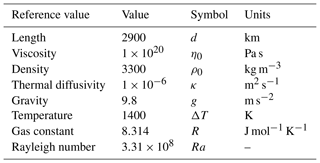Table A2Typical model element resolution was 800×160. Sub. stands for subduction.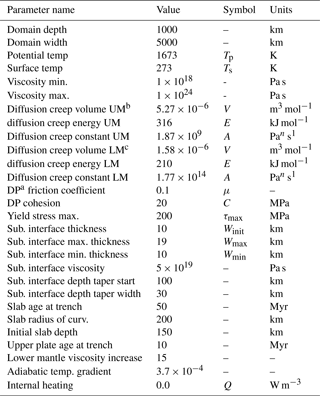Dimensional model parameters: a Drucker–Prager. b Upper mantle. c Lower mantle.

Author contributions
Author contributions.

DS and LM conceived the study and contributed equally in developing the specific methodologies discussed here. DS performed and analysed the numerical models. DS wrote the paper with input from LM.

Competing interests
Competing interests.

The authors declare that they have no conflict of interest.

Acknowledgements
Acknowledgements.

The study benefited from the authors' attendance of the CIDER summer programs in 2016 and 2017 (funded by NSF grant EAR-1135452). This work was supported by resources provided by the Pawsey Supercomputing Centre with funding from the Australian government and the government of Western Australia. This research was supported by use of the Nectar Research Cloud, a collaborative Australian research platform supported by the National Collaborative Research Infrastructure Strategy (NCRIS).

Financial support
Financial support.

This research has been supported by the Centre of Excellence for Core to Crust Fluid Systems, Australian Research Council (grant no. DP150102887). Dan Sandiford's postgraduate research at the University of Melbourne was supported by a Baragwanath Geology Research Scholarship.

Review statement
Review statement.

This paper was edited by Taras Gerya and reviewed by Hana Cizkova, Thibault Duretz, and one anonymous referee.

References

Aagaard, B., Knepley, M., and Williams, C.: PyLith v2.2.1, Computational Infrastructure for Geodynamics, https://doi.org/10.5281/zenodo.886600, 2017. a

Abers, G. A.: Seismic low-velocity layer at the top of subducting slabs: observations, predictions, and systematics, Phys. Earth Planet. Int., 149, 7–29, 2005. a, b

Agrusta, R., Goes, S., and van Hunen, J.: Subducting-slab transition-zone interaction: Stagnation, penetration and mode switches, Earth Planet. Sc. Lett., 464, 10–23, 2017. a, b, c

Alisic, L., Gurnis, M., Stadler, G., Burstedde, C., Wilcox, L. C., and Ghattas, O.: Slab stress and strain rate as constraints on global mantle flow, Geophys. Res. Lett., 37, L22308, https://doi.org/10.1029/2010gl045312, 2010. a, b

Androvičová, A., Čížková, H., and van den Berg, A.: The effects of rheological decoupling on slab deformation in the Earths upper mantle, Stud. Geophys. Geod., 57, 460–481, 2013. a

Arcay, D.: Dynamics of interplate domain in subduction zones: influence of rheological parameters and subducting plate age, Solid Earth, 3, 467–488, https://doi.org/10.5194/se-3-467-2012, 2012. a, b

Arcay, D.: Modelling the interplate domain in thermo-mechanical simulations of subduction: Critical effects of resolution and rheology, and consequences on wet mantle melting, Phys. Earth Planet. Int., 269, 112–132, 2017. a, b, c, d, e

Arnold, D. N. and Logg, A.: Periodic table of the finite elements, SIAM News, 47, 212–220, 2014. a

Arredondo, K. M. and Billen, M. I.: Coupled Effects of Phase Transitions and Rheology in 2D Dynamical Models of Subduction, J. Geophys. Res.-Sol. Ea., 2008JB005927, https://doi.org/10.1029/2008JB005927, 2017. a, b

Audet, P. and Schwartz, S. Y.: Hydrologic control of forearc strength and seismicity in the Costa Rican subduction zone, Nat. Geosci., 6, 852, 2013. a

Audet, P., Bostock, M. G., Christensen, N. I., and Peacock, S. M.: Seismic evidence for overpressured subducted oceanic crust and megathrust fault sealing, Nature, 457, 76–78, 2009. a, b

Babeyko, A. and Sobolev, S.: High-resolution numerical modeling of stress distribution in visco-elasto-plastic subducting slabs, Lithos, 103, 205–216, 2008. a

Bachmann, R., Oncken, O., Glodny, J., Seifert, W., Georgieva, V., and Sudo, M.: Exposed plate interface in the European Alps reveals fabric styles and gradients related to an ancient seismogenic coupling zone, J. Geophys. Res.-Sol. Ea., 114, B05402, https://doi.org/10.1029/2008JB005927 2009. a

Bayet, L., John, T., Agard, P., Gao, J., and Li, J.-L.: Massive sediment accretion at 80 km depth along the subduction interface: Evidence from the southern Chinese Tianshan, Geology, 46, 495–498, https://doi.org/10.1130/G40201.1, 2018. a

Bebout, G. E. and Penniston-Dorland, S. C.: Fluid and mass transfer at subduction interfaces, The field metamorphic record, Lithos, 240, 228–258, 2016. a, b, c

Becker, T. W.: Superweak asthenosphere in light of upper mantle seismic anisotropy, Geochem. Geophys. Geosy., 18, 1986–2003, 2017. a

Behr, W. M. and Becker, T. W.: Sediment control on subduction plate speeds, Earth Planet. Sc. Lett., 502, 166–173, https://doi.org/10.1016/j.epsl.2018.08.057, 2018. a, b, c

Bercovici, D.: The generation of plate tectonics from mantle convection, Earth Planet. Sc. Lett., 205, 107–121, 2003. a

Bercovici, D. and Ricard, Y.: Plate tectonics damage and inheritance, Nature, 508, 513–516, https://doi.org/10.1038/nature13072, 2014. a

Billen, M. I. and Hirth, G.: Rheologic controls on slab dynamics, Geochem. Geophys. Geosy., 8, Q08012, https://doi.org/10.1029/2007gc001597, 2007. a, b

Billen, M. I., Gurnis, M., and Simons, M.: Multiscale dynamics of the Tonga-Kermadec subduction zone, Geophys. J. Int., 153, 359–388, 2003. a

Brooks, A. N. and Hughes, T. J.: Streamline upwind/Petrov-Galerkin formulations for convection dominated flows with particular emphasis on the incompressible Navier-Stokes equations, Comput. Method. Appl. M., 32, 199–259, 1982. a

Capitanio, F., Stegman, D., Moresi, L., and Sharples, W.: Upper plate controls on deep subduction, trench migrations and deformations at convergent margins, Tectonophysics, 483, 80–92, 2010. a

Chertova, M. V., Geenen, T., van den Berg, A., and Spakman, W.: Using open sidewalls for modelling self-consistent lithosphere subduction dynamics, Solid Earth, 3, 313–326, https://doi.org/10.5194/se-3-313-2012, 2012. a

Christensen, U.: Convection with pressure-and temperature-dependent non-Newtonian rheology, Geophys. J. Int., 77, 343–384, 1984. a

Christensen, U. R.: The influence of trench migration on slab penetration into the lower mantle, Earth Planet. Sc. Lett., 140, 27–39, 1996. a

Čížková, H. and Bina, C. R.: Effects of mantle and subduction-interface rheologies on slab stagnation and trench rollback, Earth Planet. Sc. Lett., 379, 95–103, 2013. a, b, c

Čıžková, H., van Hunen, J., van den Berg, A. P., and Vlaar, N. J.: The influence of rheological weakening and yield stress on the interaction of slabs with the 670 km discontinuity, Earth Planet. Sc. Lett., 199, 447–457, 2002. a

Cloos, M. and Shreve, R. L.: Subduction-channel model of prism accretion, melange formation, sediment subduction, and subduction erosion at convergent plate margins: 1. Background and description, Pure Appl. Geophys., 128, 455–500, 1988. a, b

Duarte, J. C., Schellart, W. P., and Cruden, A. R.: How weak is the subduction zone interface?, Geophys. Res. Lett., 42, 2664–2673, 2015. a, b

Gao, X. and Wang, K.: Strength of stick-slip and creeping subduction megathrusts from heat flow observations, Science, 345, 1038–1041, 2014. a, b, c

Garel, F., Goes, S., Davies, D. R., Davies, J. H., Kramer, S. C., and Wilson, C. R.: Interaction of subducted slabs with the mantle transition-zone: A regime diagram from 2-D thermo-mechanical models with a mobile trench and an overriding plate, Geochem. Geophys. Geosy., 15, 1739–1765, https://doi.org/10.1002/2014gc005257, 2014. a, b, c

Gerardi, G. and Ribe, N. M.: Boundary-element modeling of two-plate interaction at subduction zones: scaling laws and application to the Aleutian subduction zone, J. Geophys. Res.-Sol. Ea., 123, 5227–5248, https://doi.org/10.1002/2017JB015148, 2018. a, b

Gerya, T. V., Connolly, J. A., and Yuen, D. A.: Why is terrestrial subduction one-sided?, Geology, 36, 43–46, 2008. a

Glerum, A., Thieulot, C., Fraters, M., Blom, C., and Spakman, W.: Nonlinear viscoplasticity in ASPECT: benchmarking and applications to subduction, Solid Earth, 9, 267–294, https://doi.org/10.5194/se-9-267-2018, 2018. a

Gurnis, M. and Hager, B. H.: Controls of the structure of subducted slabs, Nature, 335, 317–321, 1988. a

Hager, B. H. and O'Connell, R. J.: A simple global model of plate dynamics and mantle convection, J. Geophys. Res., 86, 4843, https://doi.org/10.1029/jb086ib06p04843, 1981. a

Hardebeck, J. L.: Stress orientations in subduction zones and the strength of subduction megathrust faults, Science, 349, 1213–1216, 2015. a, b

Hardebeck, J. L. and Loveless, J. P.: Creeping subduction zones are weaker than locked subduction zones, Nat. Geosci., 11, 60–66, 2018. a

Hirauchi, K.-i. and Katayama, I.: Rheological contrast between serpentine species and implications for slab–mantle wedge decoupling, Tectonophysics, 608, 545–551, 2013. a

Hirth, G. and Kohlstedt, D.: Rheology of the upper mantle and the mantle wedge: A view from the experimentalists, Inside the subduction Factory, 138, 83–105, 2004. a

Holt, A. F., Buffett, B. A., and Becker, T. W.: Overriding plate thickness control on subducting plate curvature, Geophys. Res. Lett., 42, 3802–3810, 2015. a

Huene, R. and Scholl, D. W.: Observations at convergent margins concerning sediment subduction, subduction erosion, and the growth of continental crust, Rev. Geophys., 29, 279–316, 1991. a

Jain, C., Korenaga, J., and Karato, S.-i.: On the yield strength of oceanic lithosphere, Geophys. Res. Lett., 44, 9716–9722, 2017. a

Karato, S.-I.: Deformation of earth materials: an introduction to the rheology of solid Earth, Cambridge University Press, Cambridge, 2012. a

Karato, S.-i. and Wu, P.: Rheology of the upper mantle: A synthesis, Science, 260, 771–778, 1993. a

Kimura, G., Yamaguchi, A., Hojo, M., Kitamura, Y., Kameda, J., Ujiie, K., Hamada, Y., Hamahashi, M., and Hina, S.: Tectonic mélange as fault rock of subduction plate boundary, Tectonophysics, 568, 25–38, 2012. a

Kincaid, C. and Sacks, I. S.: Thermal and dynamical evolution of the upper mantle in subduction zones, J. Geophys. Res.-Sol. Ea., 102, 12295–12315, 1997. a

Krien, Y. and Fleitout, L.: Gravity above subduction zones and forces controlling plate motions, J. Geophys. Res.-Sol. Ea., 113, B09407, https://doi.org/10.1029/2007JB005270, 2008. a, b

Lamb, S.: Shear stresses on megathrusts: Implications for mountain building behind subduction zones, J. Geophys. Res.-Sol. Ea., 111, B07401, https://doi.org/10.1029/2005JB003916, 2006. a

Lenardic, A. and Kaula, W.: Self-lubricated mantle convection: Two-dimensional models, Geophys. Res. Lett., 21, 1707–1710, 1994. a, b

Li, B. and Ghosh, A.: Near-continuous tremor and low frequency earthquake (LFE) activities in the Alaska-Aleutian subduction zone revealed by a mini seismic array, Geophys. Res. Lett., 44, 5427–5435, https://doi.org/10.1002/2016GL072088, 2017. a

Magni, V., van Hunen, J., Funiciello, F., and Faccenna, C.: Numerical models of slab migration in continental collision zones, Solid Earth, 3, 293–306, https://doi.org/10.5194/se-3-293-2012, 2012. a

Manea, V. and Gurnis, M.: Subduction zone evolution and low viscosity wedges and channels, Earth Planet. Sc. Lett., 264, 22–45, 2007. a

Moresi, L. and Solomatov, V.: Mantle convection with a brittle lithosphere: thoughts on the global tectonic styles of the Earth and Venus, Geophys. J. Int., 133, 669–682, https://doi.org/10.1046/j.1365-246x.1998.00521.x, 1998. a

Peacock, S. M.: Thermal and petrologic structure of subduction zones, Subduction top to bottom, American Geophysical Union, 119–133, 1996. a

Plank, T. and Langmuir, C. H.: Tracing trace elements from sediment input to volcanic output at subduction zones, Nature, 362, 739–743, 1993. a

Proctor, B. and Hirth, G.: Ductile to brittle transition in thermally stable antigorite gouge at mantle pressures, J. Geophys. Res.-Sol. Ea., 121, 1652–1663, 2016. a

Reynard, B.: Serpentine in active subduction zones, Lithos, 178, 171–185, 2013. a, b

Richards, M. A., Yang, W.-S., Baumgardner, J. R., and Bunge, H.-P.: Role of a low-viscosity zone in stabilizing plate tectonics: Implications for comparative terrestrial planetology, Geochem. Geophys. Geosy., 2, 2000GC000115, https://doi.org/10.1029/2000GC000115, 2001. a

Schellart, W. and Rawlinson, N.: Global correlations between maximum magnitudes of subduction zone interface thrust earthquakes and physical parameters of subduction zones, Phys. Earth Planet. Int., 225, 41–67, 2013. a

Shankar, V. and Kumar, L.: Stability of two-layer Newtonian plane Couette flow past a deformable solid layer, Phys. Fluids, 16, 4426–4442, 2004. a

Shreve, R. L. and Cloos, M.: Dynamics of sediment subduction, melange formation, and prism accretion, J. Geophys. Res.-Sol. Ea., 91, 10229–10245, 1986. a

Spiegelman, M., May, D. A., and Wilson, C. R.: On the solvability of incompressible Stokes with viscoplastic rheologies in geodynamics, Geochem. Geophys. Geosy., 17, 2213–2238, 2016. a

Tackley, P. J.: Self-consistent generation of tectonic plates in time-dependent, three-dimensional mantle convection simulations, Geochem. Geophys. Geosy., 1, 2000GC000036, https://doi.org/10.1029/2000GC000036, 2000. a, b

Tagawa, M., Nakakuki, T., Kameyama, M., and Tajima, F.: The role of history-dependent rheology in plate boundary lubrication for generating one-sided subduction, Pure Appl. Geophys., 164, 879–907, 2007. a

Tichelaar, B. W. and Ruff, L. J.: Depth of seismic coupling along subduction zones, J. Geophys. Res.-Sol. Ea., 98, 2017–2037, 1993. a

Trompert, R. and Hansen, U.: Mantle convection simulations with rheologies that generate plate-like behaviour, Nature, 395, 686–689, https://doi.org/10.1038/27185 1998.  a

Vannucchi, P., Remitti, F., and Bettelli, G.: Geological record of fluid flow and seismogenesis along an erosive subducting plate boundary, Nature, 451, 699–703, https://doi.org/10.1038/nature06486, 2008. a, b, c

Vannucchi, P., Sage, F., Phipps Morgan, J., Remitti, F., and Collot, J.-Y.: Toward a dynamic concept of the subduction channel at erosive convergent margins with implications for interplate material transfer, Geochem. Geophys. Geosy., 13, 2011GC003846, https://doi.org/10.1029/2011GC003846, 2012. a

Vrolijk, P.: On the mechanical role of smectite in subduction zones, Geology, 18, 703–707, 1990. a

Watts, A. and Zhong, S.: Observations of flexure and the rheology of oceanic lithosphere, Geophys. J. Int., 142, 855–875, 2000. a

Zhong, S. and Gurnis, M.: Viscous flow model of a subduction zone with a faulted lithosphere: Long and short wavelength topography gravity and geoid, Geophys. Res. Lett., 19, 1891–1894, https://doi.org/10.1029/92gl02142, 1992. a

Zhong, S. and Gurnis, M.: Mantle Convection with Plates and Mobile Faulted Plate Margins, Science, 267, 838–843, https://doi.org/10.1126/science.267.5199.838, 1995. a